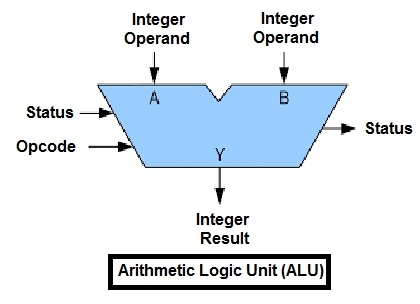# Arithmetic Logic Unit (ALU)

Inside a computer, there is an Arithmetic Logic Unit (ALU), which is capable of performing logical operations (e.g. AND, OR, Ex-OR, Invert etc.) in addition to the arithmetic operations (e.g. Addition, Subtraction etc.). The control unit supplies the data required by the ALU from memory, or from input devices, and directs the ALU to perform a specific operation based on the instruction fetched from the memory. ALU is the “calculator” portion of the computer.An arithmetic logic unit(ALU) is a major component of the central processing unit of the a computer system. It does all processes related to arithmetic and logic operations that need to be done on instruction words. In some microprocessor architectures, the ALU is divided into the arithmetic unit (AU) and the logic unit (LU).

An ALU can be designed by engineers to calculate many different operations. When the operations become more and more complex, then the ALU will also become more and more expensive and also takes up more space in the CPU and dissipates more heat. That is why engineers make the ALU powerful enough to ensure that the CPU is also powerful and fast, but not so complex as to become prohibitive in terms of cost and other disadvantages.

ALU is also known as an Integer Unit (IU). The arithmetic logic unit is that part of the CPU that handles all the calculations the CPU may need. Most of these operations are logical in nature. Depending on how the ALU is designed, it can make the CPU more powerful, but it also consumes more energy and creates more heat. Therefore, there must be a balance between how powerful and complex the ALU is and how expensive the whole unit becomes. This is why faster CPUs are more expensive, consume more power and dissipate more heat.

Different operation as carried out by ALU can be categorized as follows –

• logical operations − These include operations like AND, OR, NOT, XOR, NOR, NAND, etc.

• Bit-Shifting Operations − This pertains to shifting the positions of the bits by a certain number of places either towards the right or left, which is considered a multiplication or division operations.

• Arithmetic operations − This refers to bit addition and subtraction. Although multiplication and division are sometimes used, these operations are more expensive to make. Multiplication and subtraction can also be done by repetitive additions and subtractions respectively.